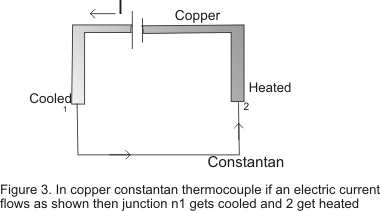# Thermal Effect of Current

## (6) Peltier Effect

• Peltier effect is named after his discover Jean Peltier who in 1934 discovered a thermo-electric effect which is converse of Seaback effect
• Peltier discovered that "when an electric current is passed through two disimilar conductor connected to form a thermo-couple ,heat is evolved at one junction and absorbed at the other end.The absorption and evolution of heat depends on the direction of flow of current• Peltier effect is entirely reversible in nature

Peltier Coefficent

• Peltier coefficent is defined as the amount of heat energy absorbed or evolved due to peltier effect at the junction of two dissimilar metals when one coulumb of charge passes through the junction
• Peltier coefficent is denoted by π
• Value of Peltier Coefficent is different for different thermo-couple .Its value also depends upon the temperature of the junction
• If q amount of charge passes through the junction then
Energy absorbed or evolved=πq
if V is the contact Potential difference
Then workdone=qV
Now heat absorbed=Workdone
So π=V
• Hence Peltier coefficent (in J/C) at a junction is numerically equal to the contact v in(Volts)

## (7) Thomson effect

• Thomson effect is related to the emf that develops between two parts of the single metal when they are at different temperature
• Thus thomson effect is the absorption or evolution of heat along a conductor when current passes through it when one end of the conductor is hot and another is cold
• If two parts of the metal are at small temperature difference dT ,themn the electric potential difference is proportional to dT dV α dT
or
dV=σdT
where σ is the constant of proportinality and is known as thomson coefficent
• Peltier coefficent and thomson coeficent are related to thermopower according to following relations
π=Ts=T(dE/dT)
and σ =-T(ds/dT)=-T(d2E/dT2)
• We have seen that all the three effects are defined in terms of three coefficent namely seaback,peltier and thomson coefficent but the basic quantity is thermo-power which is the rate of change of thermo-emf with temperature

## (8)Applications of thermoelectricity or thermo-electric effect are

• To measure temperature using thermo electric thermometer
• To detect heat radiation using thermopiles
• Thermoelectric refregerator or generator